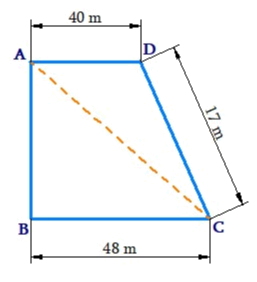# Ex.11.2 Q3 Mensuration Solution - NCERT Maths Class 8

Go back to  'Ex.11.2'

## Question

Length of the fence of a trapezium shaped field $$ABCD$$ is $$120 \,\rm{m.}$$ If $$BC = 48\,\rm{ m},\; CD = 17\,\rm{m}$$ and $$AD = 40\,\rm{ m},$$ find the area of this field. Side $$AB$$ is perpendicular to the parallel sides $$AD$$ and $$BC.$$Video Solution
Mensuration
Ex 11.2 | Question 3

## Text Solution

What is Known?

Length of the trapezium shaped field and side $$AB,\, DC,$$ and $$BC$$

What is unknown?

Area of the field and side $$AB.$$

Reasoning:

Visually area of given figure (trapezium) is sum of the area of two triangles.

Steps:

Length of the hence of a trapezium shaped field $$ABCD$$

\begin{align}&\!=\! AB + BC + CD + AD\\120\,{\rm{m }} &\!= \!AB \!+\! 48\,{\rm{m}} \!+\! 17\,{\rm{m }} \!+\! 40\,{\rm{m}}\\120\,{\rm{m}}& \!= \!AB \!+\! 105\,{\rm{m}}\\AB{\rm{ }} &= \!{\rm{ }}120\,{\rm{m }}\!-\!105\,{\rm{m}}\\ &=\! {\rm{ }}15\,{\rm{m}}\end{align}

Area of the field $$ABCD$$

\begin{align}&= \! \frac{1}{2} \! \times\! \! \begin{bmatrix}\text{Length of the}\\\text{ parallel side}\end{bmatrix}\!\! \times \! \begin{bmatrix}\text{Distance between } \\\text{two parallel sides}\end{bmatrix}\\& = \! \frac{1}{2} \! \times \! (AD \! + \! BC) \! \times \! AB\\&= \! \frac{1}{2} \! \times \! (40 \! + \! 48) \! \times \! 15\,{\rm{m}}\\& = \! \frac{1}{2} \! \times \! (88\,{\rm{m}}) \! \times \! 15\,{\rm{m}}\\& = \! 44\,{\rm{m}} \! \times \! 15\,{\rm{m}}\\&= \! 660\,{{\rm{m}}^2}\end{align}

Thus, Area of the field $$ABCD$$ is $$660\,{{\rm{m}}^2}$$.

Learn from the best math teachers and top your exams

• Live one on one classroom and doubt clearing
• Practice worksheets in and after class for conceptual clarity
• Personalized curriculum to keep up with school